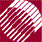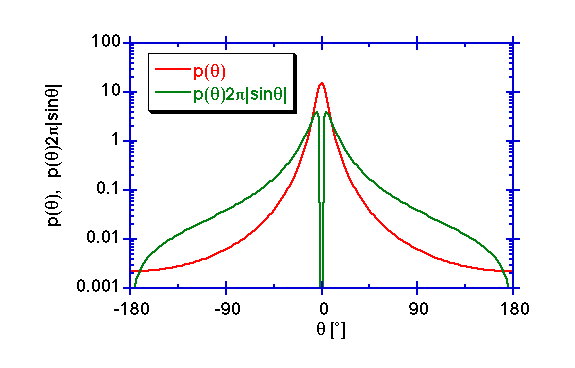ECE532 Biomedical Optics © 1998 Steven L. Jacques, Scott A. Prahl Oregon Graduate Institute

# Scattering functions

The angular dependence of scattering is called the scattering function, p(θ) which has units of [sr-1] and describes the probability of a photon scattering into a unit solid angle oriented at an angle θ relative to the photons original trajectory. Note that the function depends on only on the deflection angle θ and not on the azimuthal angle ψ. Such azimuthally symmetric scattering is a special case, but is usually adopted when discussing scattering. However, it is possible to consider scattering which does not exhibit azimuthal symmetry. The p(θ) has historically been also called the scattering phase function.

The scattering can be described in two ways:

• Plotting p(θ) indicates how photons will scatter as a function of θ in a single plane of observation (source-scatterer-observer plane). This pattern is similar to the type of goniometric scattering experiments commonly conducted.

• Plotting p(θ)2πsinθ indicates how photons will scatter as a function of the deflection angle θ regardless of the azimuthal angle ψ, in other words integrating over all possible ψ in an azimuthal ring of width dθ and perimeter 2πsinθ at some given θ. The p(θ)2πsinθ goes to zero at 0° because the azimuthal ring becomes vanishingly small at 0°. This plot is related to the total energy scattered at a given deflection angle and hence is more pertinent to the value of anisotropy.Figure depicts a typical forward-directed scattering pattern p(θ) corresponding to an experimental goniometric measurement in a single source-scatterer-observer plane, and p(θ)2πsinθ which integrates over all possible azimuthal angles ψ.